Courses

# Analytic Functions (Part - 3) - Mathematical Methods of Physics, UGC - NET Physics Physics Notes | EduRev

## Physics for IIT JAM, UGC - NET, CSIR NET

Created by: Akhilesh Thakur

## Physics : Analytic Functions (Part - 3) - Mathematical Methods of Physics, UGC - NET Physics Physics Notes | EduRev

The document Analytic Functions (Part - 3) - Mathematical Methods of Physics, UGC - NET Physics Physics Notes | EduRev is a part of the Physics Course Physics for IIT JAM, UGC - NET, CSIR NET.
All you need of Physics at this link: Physics

5. The Schroedinger Equation : In its first stages of development, the quantum theory seemed to be very far removed from the continuous theories of which the theory of analytic functions is a model, but further development resulted in a surprising change which we shall sketch here.

We shall restrict our considerations to what the physicists call the hydrogen atom, or, more precisely, the spectrum of a hydrogen atom. We shall only mention the older theory (1913-1925) due to Bohr, which was based on the analogy with the solar system : a nucleus in the center and an electron going around it and (in the last stage of the theory) spinning at the same time. There were two points of difference: in the first place, not all orbits consistent with Newton's law or with Kepler's law, were permitted, but only a discrete set of orbits; in the second place, the different spectral lines were associated not with orbits but each line was associated with two orbits ; the conception was that radiation is produced by an electron jumping from one orbit to the other.

We have here, just as at the beginning of mechanics, discontinuity in the distribution of matter, but it is accentuated by discontinuity in the set of permissible orbits and discontinuities in motion (jumps). The trend from discontinuity did not appear here until 1926 when the fundamental papers by Schroedinger were published, although it must be said that his work was not entirely independent of some ideas of his predecessors. From our point of view it is interesting to note that Schroedinger formulates his idea in the title of his papers as an attempt to interpret a certain set of numbers that appear in analyzing spectra as the set of characteristic values (Eigenwerte) of a partial differential equation. (This corresponds to the transition in mechanics from the theory of a moving point to the theory of the motion of a fluid (§3).  This partial differential equation in the simplest case is

(42)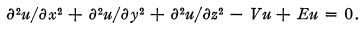Here u is a complex-valued field function, F is a given function (in the special case we are considering, the potential of the field of forces in which the electron moves), E is an undetermined constant, and the units have been chosen in such a way as to simplify the coefficients. It is surprising that equations of this type have not been considered in connection with spectra before. In acoustics the equation

(43)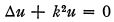was used to determine the different states of vibration of a solid. The different characteristic values, that is, the values of k for which there exist solutions of this equation compatible with certain boundary conditions depending on the geometric configuration and the physical properties of the sounding body, were taken to correspond to the different pitches of sound produced. In quantum optics, however, we have instead of boundary conditions the additional condition that the solutions should be onevalued and continuous. This should be compared with the conditions imposed on the solutions of differential equations in the derivation of the power series as a general analytic function in (f),§1. The meaning of the potential V is that by changing it we obtain different sets of characteristic values, that is, different spectra, so that it takes over some functions of the boundary conditions, because in acoustics we ordinarily get different sets of characteristic values by taking different boundary conditions.

We shall now trace, following Sommerfeld,* the series of changes that the Schroedinger equation underwent, but we shall first call attention to the fact that the connection with the theories discussed heretofore is established by the fact of the appearance of the Laplace differential operator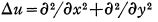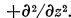. To begin with, time does not appear in the equation, but we may introduce it by reversing the process by which we got rid of d in the equation (11). We thus arrive at the equation

(44)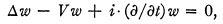which contains differentiation with respect to time but does not contain time explicitly in its coefficients; the substitution

(45)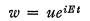brings us back exactly to the equation we had before.

This substitution is of the same nature as (12) employed before while we were obtaining a power series as a solution of the Cauchy-Riemann equations. Its justification is here the same (the equation does not contain t explicitly) f and the result is analogous; the general solution of the equation (44) appears as a series whose terms are arbitrary constants multiplied by functions of the type uneiEnt where un depend on x, y, z only, and En is a discrete set of constants.

The equation we are considering now is not quite satisfactory from the aesthetic point of view ; its form is not in keeping with the principle of relativity which for our present purposes may be formulated as the principle of treating time and space coordinates alike ; but it was found that the equation

(46)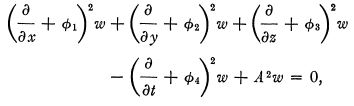which is more satisfactory in form, gives results which are only slightly different from the results obtained from the preceding equation if we set φ123 = 0, φ4= V, and give to the constants , which is the mass constant, an appropriate value. This improvement of the form without affecting much the numerical results might be compared with the transition from the original Euler equations to the equations mentioned at the end of §4.

We may say that we have now essentially a Laplace-like equation. More precisely, except for the potential φi and the mass term, we have the wave equation. Analogy suggests that we consider two things : second-degree quantities and first-order equations, of which the Laplace-like equation is a consequence.

Beginning with second-order quantities, we notice that w is a complex number and this suggests the consideration of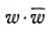. If we take for w a particular solution corresponding to one term (13) of the power series in the case of analytic function, namely,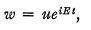we have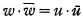and we see that t has dropped out. If we take a linear combination of such terms, however, let us say of two such terms,

(47)we get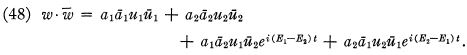The E's are interpreted as energy values, and the set of discrete values of the E's corresponds to the set of orbits in the old Bohr theory. As a result of our forming the second-degree quantitywe see then that we obtained in a natural way the fact that not the single orbits but pairs of orbits, not the separate energy values, but their differences appear in our formulas; or we may say, the transition from energy values to differences of energy values which in the Bohr theory led to the introduction of jumps, is achieved in the continuous theory by passing from the linear quantities w to the quadratic quantities. Another discontinuity is gone. It must be added that there remain apparently great difficulties in the interpretation of the coefficients ai in the preceding formulas which temper the enthusiasm which was aroused in the minds of physicists when the situation described above was first discovered by Schroedinger.

6. The Dirac Equations : On one occasion before, in pursuing the analogy between analytic functions and wave mechanics, we reversed our steps ; in analytic functions we used the substitution w = Peikδ to eliminate a variable ; in wave mechanics we used it to introduce a variable (/). We come now to another case of that nature. In analytic functions we arrive at the Laplace equation from a system of first-order equations. Here we already have a (generalized) Laplace equation and want to arrive from it at a system of first-order equations of which it is a consequence. Strictly speaking, we already have a solution of this problem. We saw that as a result of elimination of all but one component from Maxwell's system we arrive at a wave equation. It was also noted that the introduction of Maxwell's equations in optics may be considered as such a reversal from a second-order equation to a set of first-order equations. Dirac* achieved the same transition in wave mechanics, but he found another very remarkable set of first-order equations which lead to the wave equation. There are many attempts to substitute, for his equations, equations very closely related to Maxwell's equations, but it is not clear at present whether such an attempt will ever prove successful. We shall now explain Dirac's method. We strip the equation (46) of its unessential features, that is, the potentials and the mass-term. What remains we write in the form

(49)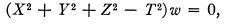where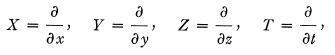and try to find a first-degree operator whose square is X2+Y2+Z2-T2. We write it in the form αX+(βY+γZ+δT, and expressing our requirement, we get

(50)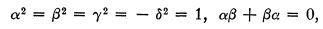etc.

In order to simplify our discussion we shall consider the two-dimensional case. The solution of the equations α2=/β2 = 1, αβ+βα = 0 in numbers is impossible, of course, but we can find matrices, viz.,

(51)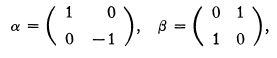which satisfy these conditions. Formally, then, the equation (αX+βY)w = 0 is equivalent to (X2 + Y2)w = 0, that is, to the equation of Laplace; or, at the least, the solutions of the first equation must satisfy the second. But how shall we interpret the operator with matrices? Among many roles in which the elements of a matrix appear, one of the most important is the role of coefficients of a linear transformation. Since we have tworowed matrices, we consider two-component vectors (u, v). Now α transforms (u, v) into (u, —v); (β transforms (u, v) into (v, u). Our equation (Xα+Yβ)w = 0 gives then exactly the CauchyRiemann equations (1).

Returning to the four-dimensional case we would expect to arrive there at four-rowed matrices; this suggests four-component vectors, and that is what Dirac and Darwin found. After the equations have been obtained, we put back the potentials and the mass constant A where they belong. It is important to note that, without potentials, the transition from one secondorder equation to four first-order equations would be only a change of form; it would not affect the physical results. It does make a great deal of difference, however, whether we put in the potentials before or after the transition. Dirac's theory fits the experimental facts still better than Schroedinger's. The transition from Schroedinger's theory to that of Dirac plays the same role as the introduction of spin in the Bohr theory. It was noted that the potentials play a role analogous to that played by boundary conditions in other cases, and it has been mentioned in connection with the introduction of Maxwell's equations in optics that the transition from second-order to first-order equations there was also important in connection with its effect on boundary conditions.

We now write out Dirac's equations essentially in the form given them by Darwin:

(52)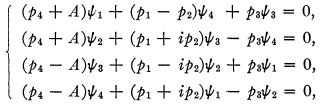where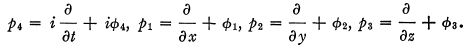In these equations we split up the complex quantities into real and imaginary parts, using the notations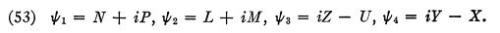Then the equations become

(54a)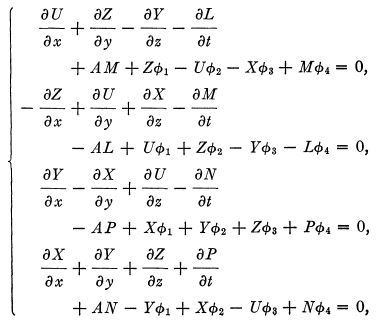(54b)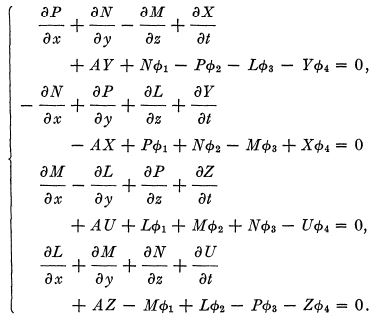The first thing we notice about these equations is that they are a generalization of Maxwell's equations (25). In fact, if we make l/ — p = Qi and also make all the potentials and the mass constant zero, we get exactly Maxwell's equations. Let us now keep U and P general but neglect the potentials and the mass constant, that is, neglect the last five terms in each equation. The resulting system is also a generalization of Maxwell's system, and therefore of the Cauchy-Riemann system (1). It has been investigated from this point of view by Iwanenko and Nikolski, who consider all the eight components of Dirac as the components of a biquaternion, and, extending the investigation of Hanni,f obtain the equations as integrability conditions, that is, write certain integrals whose vanishing is equivalent to the original equations. It may be noted that these equations do not come under the Volterra theory.

A very interesting point about these equations is that although they are relativistically invariant, (that is, if we pass to another coordinate system, we may express exactly the same thing the equations tell us by a system of equations of the same type, with different values of the components, of course) the relations between the values of components in one system of coordinates and the values in another system, that is, the transformation formulas, are not of the type considered in ordinary tensor analysis. Much has been made of this circumstance. Weyl* connected it in a very beautiful way with the theory of representation of groups. Van der Waerdenf invented a new tensor analysis, called spinor analysis, that takes care of the situation in a satisfactory way.

The situation takes a different aspect, however, when we introduce the second-order quantities. In the Schroedinger theory there was only one complex-valued component. We considered only one real-valued quadratic expression in it, namely its norm (see (48)). Now, as the result of the transition to first-order equations, we have many more components. Quite early in the development of the subject, in the first papers by Dirac and Darwin, a four-vector, two scalars, and a six-vector were introduced, whose components are quadratic in the ψ's, and are transformed according to the ordinary formulas of tensor calculus. Further investigation showed the existence of still another four-vector whose components are quadratic in the ψ's. As a result of the fact that all these components are expressed in terms of the four ψ's there exist between them many relations. Limiting our attention to the two f our-vectors, we find that they are perpendicular and of equal length.  This reminds us of analytic functions; we ask ourselves whether the divergences of these vectors vanish and find that they do, in the case when the mass constant is zero. We have thus in the Dirac theory two equal and perpendicular vectors with vanishing divergences. The theory seems then to provide a method of studying such situations, which, as we saw before (beginning of §2), involve non-linear relations, by means of linear equations, because the Dirac equations are linear. The method is based on introducing auxiliary quantities in such a way that the quadratic relations are the result of the fact that the two vectors are expressed in terms of the same auxiliary quantities. Taking this hint we find without difficulty that the components of two equal and perpendicular vectors in ordinary three-space (14) may be expressed as follows: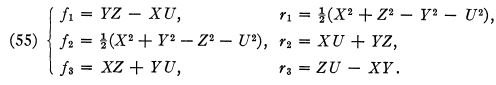If we subject these vectors to the condition (16) that their divergences should vanish, we obtain for the auxiliary quantities, X, Y, etc., a system of equations, which, although not linear, is a consequence of the linear system :which in many ways is similar to the Dirac system with potentials and mass constant neglected.

In conclusion, a word concerning the potentials. As in the other cases in which we considered second-degree quantities, we may ask the questions whether the first-degree quantities are determined by the second-degree quantities, and what differential conditions are imposed on the second-degree quantities by the differential equations to which the first-order quantities are subjected. The answer to the first question is that the firstdegree quantities are not completely determined by the seconddegree quantities, but are determined to within a variable angle, just as (u, v) are not determined by u2+v2, or r and ƒ by E. In answering the second question, we have to eliminate therefore this variable angle, just as in the cases we had before. Without entering into details we may state that if we start with Dirai s equation s withou t potential s we obtai n in a wa y analogou s to that by which we obtained (10) and (41), a system of exactly the form of the Dirac equations with potentials, except that the φ's are the derivatives of the arbitrary angle.

Offer running on EduRev: Apply code STAYHOME200 to get INR 200 off on our premium plan EduRev Infinity!

159 docs

,

,

,

,

,

,

,

,

,

,

,

,

,

,

,

,

,

,

,

,

,

,

,

,

;